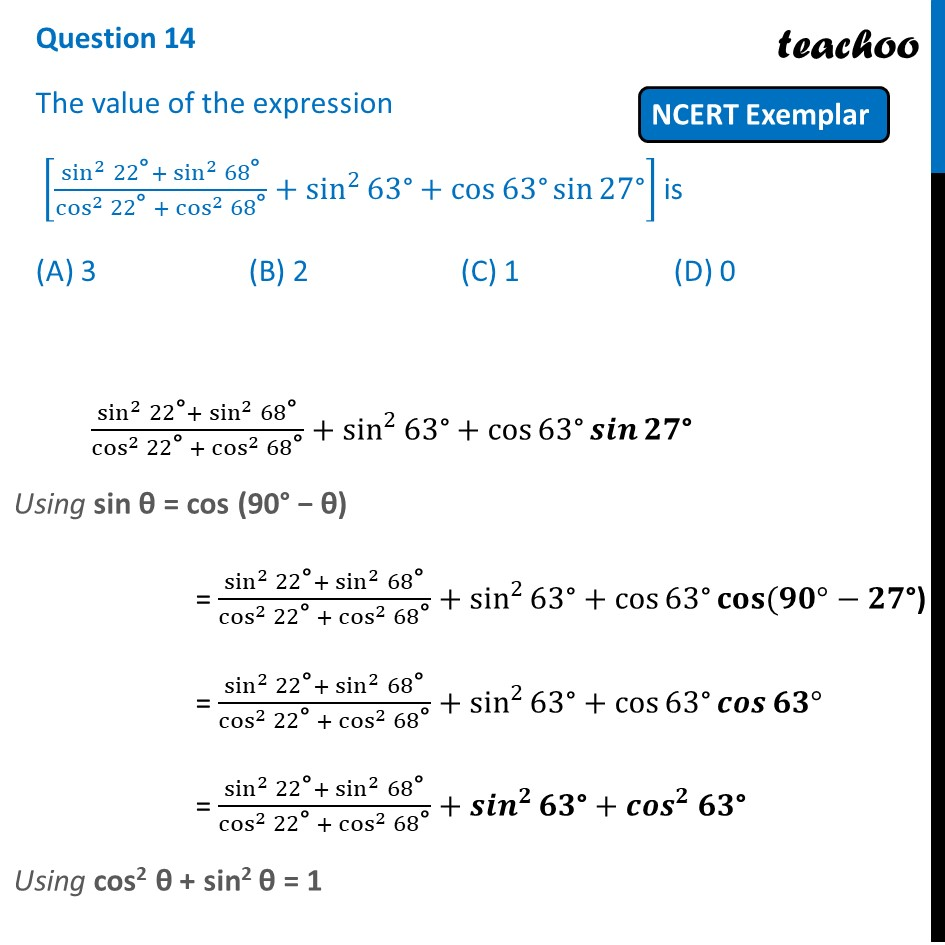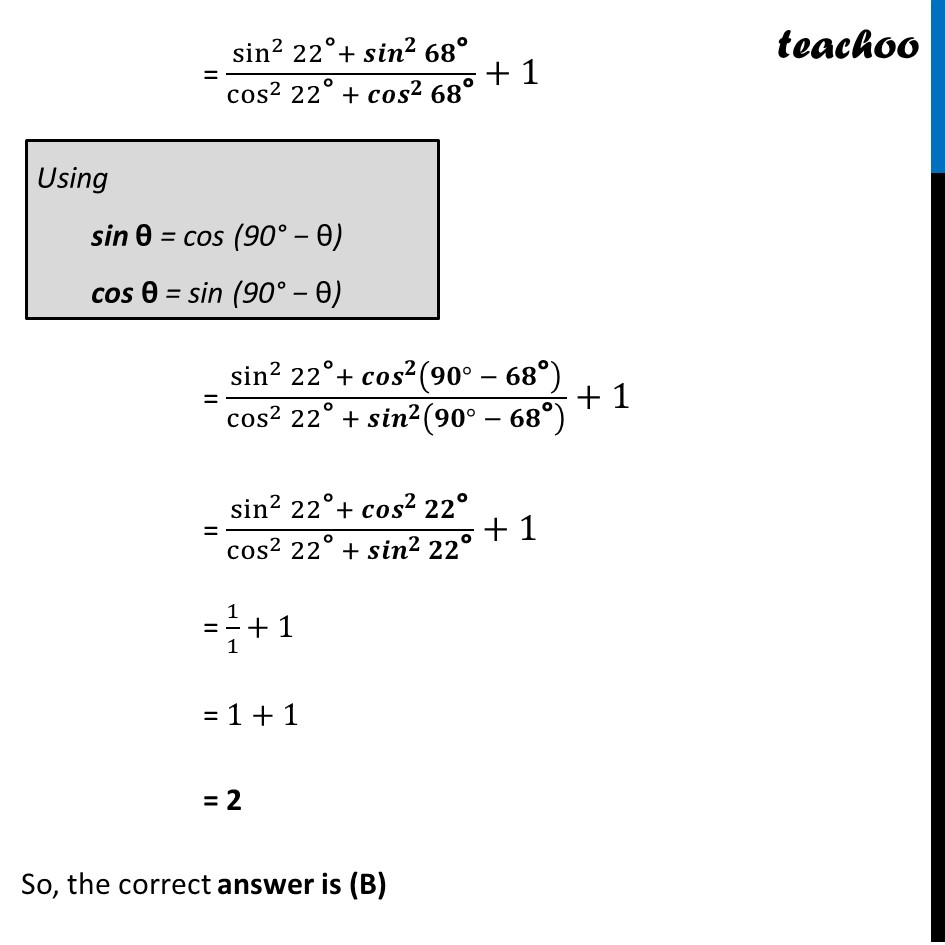NCERT Exemplar - MCQ

Chapter 8 Class 10 Introduction to Trignometry
Serial order wise

## (A) 3  (B) 2  (C) 1  (D) 0Learn in your speed, with individual attention - Teachoo Maths 1-on-1 Class

### Transcript

Question 14 The value of the expression [(sin^2⁡22"°" + sin^2⁡68"°" )/(cos^2⁡〖22"°" 〗+ cos^2⁡68"°" )+sin^2⁡〖63"°" +cos⁡〖63"°" sin⁡27"°" 〗 〗 ] is (A) 3 (B) 2 (C) 1 (D) 0 (sin^2⁡22"°" + sin^2⁡68"°" )/(cos^2⁡〖22"°" 〗+ cos^2⁡68"°" )+sin^2⁡〖63"°" +cos⁡63"°" 𝒔𝒊𝒏⁡𝟐𝟕"°" 〗 Using sin θ = cos (90° − θ) = (sin^2⁡22"°" + sin^2⁡68"°" )/(cos^2⁡〖22"°" 〗+ cos^2⁡68"°" )+sin^2⁡〖63"°" +cos⁡〖63"°" 𝐜𝐨𝐬⁡〖(𝟗𝟎°−𝟐𝟕"°)" 〗 〗 〗 = (sin^2⁡22"°" + sin^2⁡68"°" )/(cos^2⁡〖22"°" 〗+ cos^2⁡68"°" )+sin^2⁡〖63"°" +cos⁡〖63"°" 𝒄𝒐𝒔⁡〖𝟔𝟑°〗 〗 〗 = (sin^2⁡22"°" + sin^2⁡68"°" )/(cos^2⁡〖22"°" 〗+ cos^2⁡68"°" )+〖𝒔𝒊𝒏〗^𝟐⁡〖𝟔𝟑"°" +〖𝒄𝒐𝒔〗^𝟐⁡𝟔𝟑"°" 〗 Using cos2 θ + sin2 θ = 1 (sin^2⁡22"°" + 〖𝒔𝒊𝒏〗^𝟐⁡𝟔𝟖"°" )/(cos^2⁡〖22"°" 〗+ 〖𝒄𝒐𝒔〗^𝟐⁡𝟔𝟖"°" )+1 = (sin^2⁡22"°" + 〖𝒄𝒐𝒔〗^𝟐⁡(𝟗𝟎° − 𝟔𝟖"°" ))/(cos^2⁡〖22"°" 〗+ 〖𝒔𝒊𝒏〗^𝟐⁡(𝟗𝟎° − 𝟔𝟖"°" ) )+1 = (sin^2⁡22"°" + 〖𝒄𝒐𝒔〗^𝟐⁡𝟐𝟐"°" )/(cos^2⁡〖22"°" 〗+ 〖𝒄𝒐𝒔〗^𝟐⁡𝟐𝟐"°" )+1 = 1+1 = 2 So, the correct answer is (B)